Reach Us+44-1522-440391
A Stochastic Segmentation Model for Recurrent Copy Number Alteration Analysis | OMICS International
Journal of Biometrics & Biostatistics

Like us on:

All submissions of the EM system will be redirected to Online Manuscript Submission System. Authors are requested to submit articles directly to Online Manuscript Submission System of respective journal.

# A Stochastic Segmentation Model for Recurrent Copy Number Alteration Analysis

Haipeng Xing* and Ying Cai

Applied Mathematics and Statistics, State University of New York, Stony Brook, NY 11794, USA

*Corresponding Author:
Haipeng Xing
Applied Mathematics and Statistics
State University of New York
Stony Brook, NY 11794, USA
E-mail: [email protected]

Received date: February 06, 2015; Accepted date: May 11, 2015; Published date: May 18, 2015

Citation: Xing H, Cai Y (2015) A Stochastic Segmentation Model for Recurrent Copy Number Alteration Analysis. J Biom Biostat 6:221. doi: 10.4172/2155-6180.1000221

Copyright: © 2015 Xing H, et al. This is an open-access article distributed underthe terms of the Creative Commons Attribution License, which permits unrestricted use, distribution, and reproduction in any medium, provided the original author and source are are credited.

Visit for more related articles at Journal of Biometrics & Biostatistics

#### Abstract

Recurrent DNA copy number alterations (CNAs) are key genetic events in the study of human genetics and disease. Analysis of recurrent DNA CNA data often involves the inference of individual samples’ true signal levels and the crosssample recurrent regions at each location. We propose for the analysis of multiple samples CNA data a new stochastic segmentation model and an associated inference procedure that has attractive statistical and computational properties. An important feature of our model is that it yields explicit formulas for posterior probabilities of recurrence at each location, which can be used to estimate the recurrent regions directly. We propose an approximation method whose computational complexity is only linear in sequence length, which makes our model applicable to data of higher density. Simulation studies and analysis of an ovarian cancer dataset with 15 samples and a lung cancer dataset with 10 samples are conducted to illustrate the advantage of the proposed model.

#### Keywords

Categorical states; Hidden Markov models; Multiple change-points; Recurrent CNAs

#### Introduction

Copy number alterations (CNAs) are key genetic events in the development and progression of numerous human diseases. Recent advances in high density microarray technologies enable high-throughput genome-wide profiling of DNA copy number; see [1,2]. Using the array-based comparative genomic hybridization (array-CGH) technology, the average genomic DNA copy number at thousands of locations linearly ordered along the chromosomes can be quantitatively measured . Since cancer genes are more likely to be found in common or recurrent regions in the sequence of CNAs across patients of the same cancer , one is more interested in finding recurrent CNA regions that consist of continuous probes and show evidence of alteration in some samples .

During the past years, a large number of computational and statistical methods have been developed to locate the recurrent CNA regions across samples, see reviews and comparisons of these methods in [5,6]. Most of these methods involve a two-step procedure, in which the first step is to identify the gain and loss regions in individual samples and the second step is to make inference on recurrent regions based on a threshold for occurrence frequencies. Examples of these approaches can be found in [7-14]. As the first steps of these approaches require segmentation of individual samples, they may strengthen or weaken some important information in recurrent regions. In contrast to twostep methods, one-stage approaches analyze raw data directly and avoid the information change in the two-step process. Recently, several statistical approaches have been proposed along this line, including score-based approach [15,16] hierarchical hidden Markov model , Bayesian hidden Markov model  kernel smoothing methods [19,20] analysis of variance approach, and likelihood-based test for simultaneous change-points . Most of these methods involve a hard segmentation procedure. However, for complex alteration profiles across samples, identified recurrent CNA regions vary greatly. This indicates that hard segmentation procedures may be difficult for identification of recurrent regions, and instead, an inference procedure on the probability of recurrent regions might be necessary.

In this paper, we propose for the analysis of recurrent CNAs a stochastic segmentation model and associated inference framework.

The proposed model has a hierarchical hidden Markov structure which make the inference framework associated to our model possess attractive statistical and computational properties. The hierarchical hidden Markov structure in our model is similar to that in Shah et al. , but our model allows different “quantitative” states conditional on a given “categorical” state, while the model in Shah et al. , assumes all “quantitative” states are same for a “categorical” state. Specifically, we assume a finite state hidden Markov chain for (categorical) states of recurrent regions across samples, and then conditional on the categorical state, signal levels (or “quantitative states”) of CNAs in each sample follow a sample-specific continuous state hidden Markov chain. As a working model, although these assumptions seem to complicate for obtaining an inference procedure, they actually provide us more flexibility to model the non-simultaneity feature of break points across samples and yields explicit recursive formulas for posterior distributions of hidden “categorical” states (i.e., the recurrent CNA region) and sample-specific “quantitative” states (i.e., the signal levels of CNAs in individual samples) at each probe, whereas the model in Shah et al. , has to rely on Monte Carlo simulations.

Our stochastic segmentation model assumes that the log fluorescence ratios ylt for sample l∈ {1, . . . , L} measured through the array-CGH technology follow that yltlt + σllt (t=1, . . . , T), in which θlt are independent standard normal random variables, and θlt are piecewise constant whose values at location t follow a prior distribution that depends on a hidden Markov chain st with three categorical states (gain, baseline 0 or loss). In this specification, θit and st represent the true signal levels of CNAs in sample l and the gain-loss states across the L samples at location t. When st shifts from one categorical state to another, signal levels (or quantitative states) θlt in sample l jump to a new state, whose prior distribution depends on st, hence θlt may be different from the quantitative states whose corresponding categorical states are same as st. Making use of this specific hierarchical model structure, we compute the posterior distributions of θlt and sl based on explicit recursive formulas that are derived using forward and backward filters of the hidden Markov model. The forward-backward filters in our model can be considered as a non-trivial extension of the Baum-Welch algorithm and similar to those developed by [22-24]. The difference of our forward-backward filters from previous work is that the hidden categorical and quantitative states in our model have a hierarchical structure and the top layer hidden states become a finitestate Markov chain. As the problem of locating recurrent CNA regions intrinsically involves much computation, to reduce the computational complexity of our inference procedure, we further consider a bounded complexity mixture approximation scheme so that the computational complexity becomes linear. Another discussion we have made is that, since all model hyper parameters are unknown in real applications, we propose to estimate all hyper parameters by an expectationmaximization (EM) algorithm.

The rest of the paper is organized as follows. Section 2 provides the model details and develops an inference procedure. It also discusses some computational issues and proposes a bounded complexity mixture approximation scheme and a hyper parameter estimation algorithm. Section 3 shows the performance of our model and associated inference procedure via intensive simulation studies. Section 4 analyzes two groups of CNA data, one is on ovarian cancer based on 15 samples and the other is on lung cancer based on 10 patients. We identify the recurrent CNA regions related to those cancers and demonstrate that our result is consistent with that in current medical studies. Section 5 provides some conclusive remarks.

#### A Stochastic Segmentation Model

Model specification

We consider the problem of analyzing DNA copy number profiles from multiple distinct biological samples {ylt: 1 ≤ l ≤ L, 1 ≤ t ≤ T }, where ylt is the observed log fluorescence ratio at location t of sample l, T is the number of probes, and L is the number of samples. To estimate recurrent signals, we assume the following model for ylt:

yltlt + σllt,     (1)

in which ǫlt are independent Gaussian random errors with mean 0 and variance 1, σl2 are sample-specific error variances and {θlt} is the true signal level of CNA of sample l at location t. Since we want to find recurrent regions across all L samples, we assume that recurrent regions can be represented by a “master” sequence of categorical states {st}, where st ∈ {G, O, S} (gain, baseline 0, loss) is an irreducible hidden Markov chain with probability transition matrix P=(pij) and stationary distribution π. Then given the master sequence {st}, the dynamics of θlt in sample l is expressed as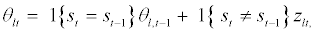(2)

in which zlt are independent normal variables with mean z(l,st) and covariance v(l,st) .

In the above model assumption, the existence of stationary distribution π could define us a reversed chain for {st}, and it further implies that the Markov chain {θlt} has a stationary distribution. Moreover, if we further assume that θl0 is initialized at the stationary distribution, {θlt} become a reversible Markov chain, which provides substantial simplification for the smoothing estimates of {θlt} and st. We should note that this assumption is to simplify the estimation procedure. It may not reflect the real situation since the probability of amplification or deletion might be different across the whole chromosome.

The assumption that the master sequence of states is common across all the samples may not be necessarily true in practice, and it is only an approximation for the fact that most samples share a unique profile signature. This assumption is used in some models to obtain an estimation procedure with reasonable computational complexity for identifying recurrent CNAs; see Shah et al. The assumption also implies that the model is not suitable for a class of samples that consists of several disease subclasses with each subclass having a unique profile signature. For sample with disease subclass, we need to know the information about disease subclasses before applying the above model. Furthermore, assumption (2) indicates that signal levels θlt with same categorical states could be different.

Filtering estimate

Let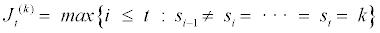be the most recent location where st switches to state k from other states prior or equal to t. Denote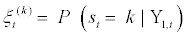and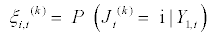for 1 ≤ i ≤ t and 1 ≤ k ≤ K=3, in which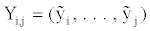and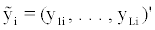. By definition, we have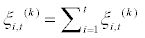. Then given Y1,t and the most recent switching location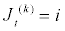, the conditional distribution of θlt is given by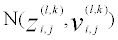, where for j ≥ i,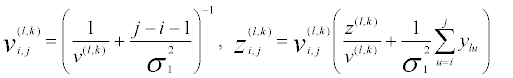(3)

conditional distribution of θlt given Y1,t becomes a mixture of normal distributions: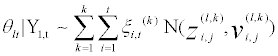(4)

Let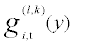and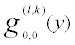denote the density function of theand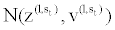distributions at y, respectively. Making use of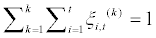and model assumption (1), Web Appendix A show that the conditional probabilities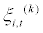can be determined by the following recursions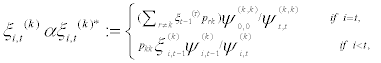(5)

where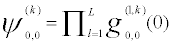and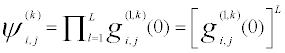. Specifically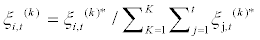. Then by (4),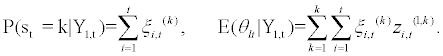(6)

Smoothing estimate

Our model assumptions imply that the stationary distribution of θlt exists and is given by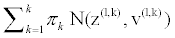. This indicates that, if θlt is initialized at its stationary distribution, its time-reversed Markov chain can be defined. This substantially simplifies the smoothing estimates of θlt. Actually, it further implies that {θlt} is a reversible Markov chain, so we can reverse time and obtain a backward filter that is analogous to (4):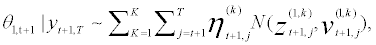(7)

Where the mixture weight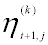is given by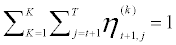and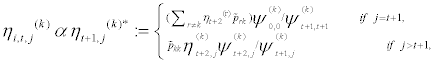(8)

in which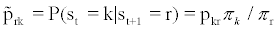is the transition probability of the reversed chain of {st}. Since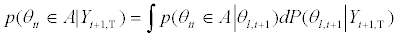, it follows from (8) and the reversibility of {θt} that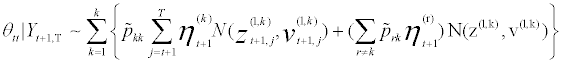(9)

Next, we shall use Bayes’ theorem to combine the forward filter (4) with its backward variant (9) to estimate θlt given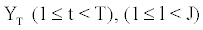, which is expressed as the following mixture of normal distributions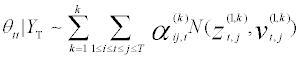(10)

In which the mixture weights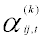are posterior probabilities explained below. Consider the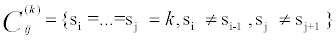, Web Appendix A shows that, for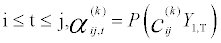andan be calculated recursively as follows: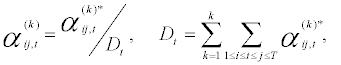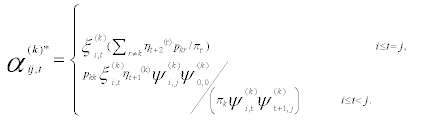(11)

Therefore, from (10), it follows that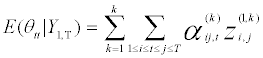(12)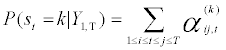(13)

Figure 1 provides a schematic explanation for the above algorithm. For location t, the algorithm first decomposes conditional distributions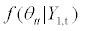and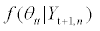based on the most recent switching location of st before and after t, then use Bayes theorem to combine these twodistributions and obtain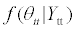Bounded complexity mixture approximations and hyper parameter estimation

The number of mixture weights in the above discussion increases dramatically with t (or n), resulting in rapidly increasing computational complexity and memory requirements in estimating θlt as t keeps increasing. To address the issue of computational efficiency, we follow Lai and Xing and use a bounded complexity mixture (BCMIX) approximation procedure with linear computational complexity; see Web Appendix B for details of the algorithm.

The inference procedures involve the hyper parameters p, probability transition matrix P, and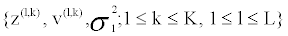. In practice, these [(K − 1) K + (2K + 1) L + 1] parameters are unknown and should be replaced by their estimates. We consider an EM algorithm to estimate all hyper parameters with the details given in Web Appendix C.

In practical applications, we should also notice that the three categorical states in the above model are exchangeable; hence the categorical states st could be very difficult to identify. A remedy for this is to replace the normal priors for θlt by truncated priors, then the filtering and smoothing formulas in Sections 2.2 and 2.3 needs to be modified somewhat. Specifically, the normal distribution in conditional distribution (10) needs to be replaced by corresponding truncated normal distributions. Another way to mitigate the identification issue is to put constraints on hyper parameters. For example, a prior normal distribution with smaller variances could limit the estimated quantitative signals staying around its prior mean, so the distinction between the categorical states becomes clearer.

Simulation Studies

We now perform intensive simulations to evaluate the performance of the proposed model and inference procedure from frequentist and Bayesian viewpoints. To demonstrate the performance, we consider two measures for different purpose. One measure is mean square error (MSE), which provides the mean errors between the estimates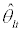and the true θlt, i.e.,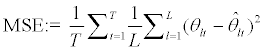and the other is mean identification rate (IR), which evaluates the accuracy of our inference on the hidden states st. As our model only computes the posterior probability of st given Y1,T , we estimate the state of location t as the one which maximizes the posterior probability of being in categorical state k, i.e.,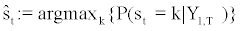With the above estimate, we define the mean identification ratio as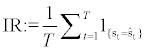We first evaluate the performance of BCMIX estimates in the frequentist setting by considering the following four cases of hidden state {st} with K=3.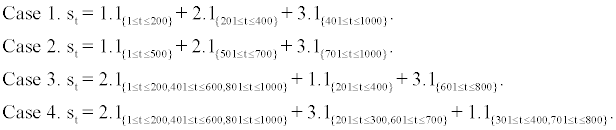Given the above {st}, we generate θlt by assuming θlt ∼ N (z(l, st ), v(l, st )) with (z(l,1), z(l,2), z(l,3) )=(1, 0, −1), v(l,1)=v(l,2)=v(l,3)=0.22 (hence the standard deviation is about 0.47). We further assume L=10, T=1000, and generate observations ylt by (1) and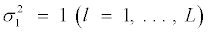. We then use the EM algorithm to estimate the hyper parameters and compute the BCMIX estimates with (M, m)=(10, 5), (20, 10), (30, 15) and (40, 20). For comparison purpose, we also compute oracle estimate which assume {st} is known. We then run such simulation for each case 500 times, and summarize the MSE of two estimates and corresponding standard errors (in parentheses) in Web Table 1. We can see that the oracle and BCMIX estimates are quite similar, and the difference among BCMIX estimates with different (M, m) are quite small. Therefore, we will use BCMIX estimate with (M, m)=(20, 10) in the following discussion.

We then evaluate the performance of the inference procedure under our model assumption. Let K= 3, (z(l,1), z(l,2), z(l,3))=(1, 0, −1), v(l,1)=v(l,2)=v(l,3)=0.16, and σ12 = 1 for 1 ≤ l ≤ L=10. The probability transition matrix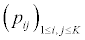of {st} is assumed to follow nine scenarios. Specifically, for Scenarios , k= 1,2,3,.....,5, we let pij=0.001×2k−1 for 1 ≤ i ≠ j ≤ 3. For Scenario S6, (p12, p13, p21, p23, p31, p32)=(0.002, 0.001, 0.002, 0.002, 0.001, 0.002). For Scenario S7, (p12, p13, p21, p23, p31, p32)=(0.004, 0.001, 0.004, 0.004, 0.001, 0.004). For Scenario S8, (p12, p13, p21, p23, p31, p32)=(0.001, 0.002, 0.001, 0.001, 0.001, 0.001). For Scenario S9, (p12, p13, p21, p23, p31, p32)=(0.001, 0.004, 0.001, 0.001, 0.001, 0.001). For each scenario, we first generate samples of different lengths with T=3000, 5000, 7000, then use the proposed EM algorithm to estimate the hyper parameters and estimate θit and P (st|Y1,T ). Web Tables 2 and 3 summarizes the MSE and IR of our estimate, and also provided in parentheses are the corresponding standard errors based on 500 simulations in each cell. We can see that the MSE are very small, and the IR is all larger than 84%.

Since data generation procedures in above studies do not deviate from our model assumption too much, to show the variability of our model, we also evaluate the performance of our algorithm on the data in Willenbrock and Fridly and , which are generated from a completely different procedure, and compute the MSE between the estimatesand the true signals θlt. The MSE of 100 datasets with 20 samples and each sample with 500 clones on Chromosome 1 is 0.011 with standard error 5.89e-4, indicating the estimates for signals in individual samples is still pretty good. Web Figure 1 demonstrates a randomly selected simulated ylt andfor 20 samples.

We next compare our model to the hierarchical hidden Markov model (HMM) in Shah et al. . Specifically, we estimate all hyper parameters by the EM algorithm, and then fit the hierarchical HMM model to the simulated data generated in Scenarios S1-S9. Since Shah et al. assume the signal levels θlt of individual CNAs follow a normal distribution with the mean and variance depending on the hidden state st directly; it implies that the individual signal levels are fixed within the same segment of recurrent CNA regions. This is different from our model which allows signal levels θlt of sample l have different values at different locations t even if their categorical states st are same, which is more realistic in practice. Furthermore, our algorithm avoids the use of Markov Chain Monte Carlo algorithm, hence computationally is very fast. We run all simulations on a desktop with Intel core i5- 3210M and 4G memory, for each simulation of 10 samples with sample length T=3000, 4000, 5000 and 6000, our algorithm takes about 2.8-6 seconds to obtain the estimates for θlt and sl , while the hierarchical HMM model takes over 10-20 minutes to get its estimates. Web Table 3 summarizes the identification ratios and the corresponding standard errors (in parentheses) using Shah et al.’s model for different settings. Each cell is based on 100 simulations. We shall note that the hidden states st in our setting are very close to each other due to the large signalto- noise ratios, hence it is not easy to make a correct state calling. The identification ratios of the hierarchical HMM are very good (all their ratios are about 70%), but they are typically smaller than ours.

#### Real data studies

Analysis for Ovarian cancer data

Ovaries are reproductive organs that produce eggs in women, and ovarian cancer is the fifth leading cause of cancer death in women. Ovarian cancers display a high degree of complex genetic variations. The existing literature show that the most frequently affected chromosomes in ovarian cancer are chromosome 1, 8, and 17. We use our model to analyze the copy number alteration (CNA) data for Ovarian serous cystadenocarcinoma (OV) based on Array based-CGH technology. The data in our analysis, consisting of the CNAs from 15 OV cancer patients, were published on April 1st, 2010 in the Cancer Genome Atlas (TCGA) database (http://cancergenome.nih.gov/). We analyze the whole 23 chromosomes. Since the existing literature shows that the most frequently affected chromosomes in ovarian cancer are chromosomes 1, and 17, we only present our result on these two chromosomes.

There are 55,274 and 20,009 probes on chromosomes 1 and 17. Let k=1, 2 and 3 denote amplification, baseline and deletion, respectively. We first use the proposed model and inference procedure to estimate the hyper parameters, and then the signal levels θlt and probability of st for chromosomes 1 and 17. We run our model on a desktop with Intel core i5-3210M and 4G memory, and it takes 223 and 109 seconds for chromosomes 1 and 17, respectively. The results are summarized in Web Figures 2 and 3, respectively. We can see that our procedure analyzes all samples and estimates signal θlt for each sample simultaneously, which avoid the weakness of two-stage analysis. As our interest here is the recurrent CNA region, we now focus on the estimated probabilities of st, which are plotted in Figure 2. Those estimated probabilities indicate that the recurrent copy number amplifications involve regions 1p34.2, 1p12, 1q23.2 and 1q42.3, and deletions involve regions 1p36.33, 1p36.21, 1p36.13, 17p11.2 and 17p12. Well known tumor suppressor genes TP73 (1p36.33), TP53 (17p13.1), BRCA1 (17q21), oncogene MYCL1 (1p34.2), and transcription factors RAI1 (17p11.2), SREBF1 (17p11.2) are found recurrent regions of copy number variants. Our results are consistent with earlier studies [26-28]. It is important to note that for chromosome 17, we focus on detecting the recurrent regions of copy number deletions, since the most common alterations for serous histology of OV cancer are deletions of 17p [26,28,29].

Figure 2: Estimated P (st=k|Y1,T) of chromosomes 1 (The top two) and 17 (The bottom two) for k=amplification (The 1st and 3rd panels) and deletion (The 2nd and 4th panels).

There are totally 178 and 136 unique known genes involving in recurrent CNA regions for chromosomes 1 and 17 respectively. These known genes are subjected to pathway exploration using the Ingenuity Pathway Analysis (IPA) software (Ingenuity Systems, Redwood City, CA). Significantly enriched pathways with Fishers exact P-values less than 0.05 are listed in this bar plot as shown in Figure 3. Yellow square line in the figure represents ratio which is the number of focus genes in the pathway divided by the total number of genes that make up that pathway. For chromosome 1, most of the pathways are related to cancer. Notably, as listed on the 7th, the breast cancer signaling was found enriched. Furthermore, a few hormone metabolism pathways are involved, which includes PXR/RXR activation, Estrogen receptor signaling and Aldosterone signaling in Epithelial Cells. This is consistent with current knowledge of disrupted hormone metabolism pathways as important causal factors in breast cancer [30-32]. In addition, a few important cellular pathways are revealed: The NRF2- mediated oxidative stress response turns out to be most significantly changed in the list, which has been related to breast cancer. The G-protein signaling pathway, which is well-known to be related to cancer, is listed on the second. A basic transcription factor related pathway is ranked on the 3rd. And listed on the 4th, Ubiquitination regulates degradation of cellular proteins by the ubiquitin proteasome system, controlling a proteins half-life and expression levels. A change of ubiquitination activity is associated with ovarian tumorigenesis, so the protein ubiquitination pathway might be involved in breast ovarian progression. Finally, one of the most important developmental pathway in mammals, Notch signaling also known to play a role in cancer . For chromosome 17, the pathway enrichment resultreveals some biological mechanisms and pathway changes involved in ovarian cancer. First obviously, the ovarian cancer signaling pathway was found enriched. Particularly, the GADD45 and p53 signaling pathways are enriched. Both these two factors, especially p53, are well established tumor suppressor proteins. More importantly, almost half of the pathways are basic and critical cellular processes such as DNA repair, cell cycle regulation and apoptosis. Changes in these pathways indicate severe disruptions of normal cellular functions. This could be either the cause or the result of cancer.

Analysis for lung cancer data

There are two main types of lung cancer, small cell lung carcinoma (SCLC) and non-small cell lung carcinoma (NSCLC) . NSCLC is the most common type of lung cancer, accounting for about 85% of total lung cancers. NSCLC is mainly comprised of adnenocarcinoma, squamous cell carcinoma and large cell carcinoma. About 30% of lung cancers are squamous cell carcinoma. Previous cancer studies have revealed that multiple tumor suppressor genes are involved in deletions at multiple chromosomal regions in lung carcinogenesis, and the most frequent deletions in lung cancer tissues are at chromosome 3, 13 and 17.

We use our model to analyze the CNA data for Lung squamous cell carcinoma (one type of non-small cell lung cancer) based on Array based-CGH technology. The data used in our study, consisting of the CNAs from 10 cancer patients, were published on October 22nd, 2010 in the Cancer Genome Atlas (TCGA) database (http://cancergenome. nih.gov/). We analyze the whole 23 chromosomes. Since the existing literature shows that the most frequently affected chromosome in this type of lung cancer is chromosomes 17, we present our result on chromosome 17.

There are totally 13,575 probes on chromosome 17. Let k=1, 2 and 3 denote the amplification, baseline and deletion. We first use the proposed model and inference procedure to estimate hyper parameters and then fit the model to the data. We run our model on a desktop with Intel core i5-3210M and 4G memory, and it takes 30 seconds for chromosome 17. The estimated signal levels θlt for chromosomes 17 are summarized in Web Figure 4. We can see that our procedure analyzes all samples and estimates signal θlt for each sample simultaneously, which avoids the weakness of two-stage analysis. As our interest here is the recurrent CNA region, we now focus on the estimated probabilities of st, which are plotted in Figure 4. Those estimated probabilities indicate that the recurrent copy number amplifications at long arm of chromosome 17, which contains the oncogene ERBB2 at 17q12. Two regions of deletion can be found at short arm and long arm of chromosome 17 respectively, which contain the well-known tumor suppressor genes TP53 at 17p13.1, BRCA1 and CRHR1 at 17q21.31. Our results are consistent with earlier studies [35,36].

Figure 4: Estimated P (st=k|Y1,T) of chromosomes 17 for k=amplification (The 1st panels) and deletion (The 2nd and 4th panels).

#### Conclusion

We have developed a stochastic segmentation model and an associated inference procedure for recurrent CNA data. The model implies explicit recursive formulas for both the posterior distribution of individual samples’ signal levels and the probabilities of the crosssample recurrent events at each probe. This further suggests the estimate of the recurrent states of CNAs. To speed up the computation for practical purpose, an approximation to the exact explicit formulas is developed, and the computational complexity is reduced to linear order. Estimation of hyper parameters involves an explicit EM algorithm which is described in the Web Appendix D.

In Section 4, we have analyzed two real datasets to illustrate the application of our model. In particular, we identify the recurrent CNAs regions using the copy number data for ovarian serous cystadenovarcinoma and non-small lung cancer carcinoma that are produced by the array-CGH technology. The estimated CNA regions by our model are consistent with the biological discovery in medical study. For ovarian serous cystadenovarcinoma, we further perform a canonical pathway analysis to evaluate our result, and find our pathway enrichment results yield significant pathways and most of them are cancer related pathways. Our result based on chromosomes 1 and 17 already reveals certain biological mechanisms and pathway changes involved in ovarian cancer. These facts demonstrate that our model can successfully capture recurrent CNA regions and generate promising results in biological context.

#### Acknowledgements

The first author was supported by National Science Foundation DMS-0906593 and DMS-1206321. We thank gratefully the associate editor and two anonymous referees for their constructive comment on how to improve this paper.

#### References

Select your language of interest to view the total content in your interested language

### Article Usage

• Total views: 11734
• [From(publication date):
April-2015 - Aug 20, 2019]
• Breakdown by view type
• HTML page views : 7978
• PDF downloads : 3756

## Post your commentCan't read the image? click here to refresh
###### Peer Reviewed Journals

Make the best use of Scientific Research and information from our 700 + peer reviewed, Open Access Journals

International Conferences 2019-20

Meet Inspiring Speakers and Experts at our 3000+ Global Annual Meetings

Top